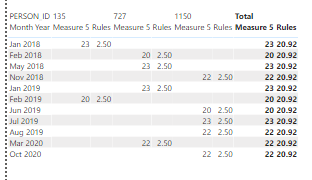cancel
Showing results for
Did you mean:Helper V

## Subtract Measure Values from Column

Is there a way to subtract column values [Rules] from a measure?

My measure which gives me number of working days in a month is:

`Measure 5 = VAR _Month = CALCULATE(COUNT(DimDate[Days in Month]),FILTER(DimDate,'DimDate'[WorkingDays] <>BLANK()))VAR _lastday= COUNTROWS( FILTER(DimDate,'DimDate'[WorkingDays] <>BLANK()))RETURN IF ( ISBLANK ( [AbsentByDay] ), BLANK (), _lastday )`

Thanks,

https://www.dropbox.com/s/vojq25pkazt4lmj/Sample5.pbix?dl=0

3 REPLIES 3Helper V

@amitchandak I think I have it here:

`Measure 9 = IF(HASONEVALUE('Employee Absence Full Table'[Rules]),[Measure 5],SUMX(ALLSELECTED('Employee Absence Full Table'),[Measure 5]))- SUMX(ALLSELECTED('Employee Absence Full Table'[Rules]),'Employee Absence Full Table'[Rules])`
But I end up with this, is there a way to just remove the values if measure 5 is blank?Super User

@Niiru1 , The output will be measure not column.

Can you share output in table format?Helper V